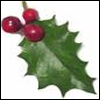#### You may also like### Giant Holly Leaf

Find the perimeter and area of a holly leaf that will not lie flat (it has negative curvature with 'circles' having circumference greater than 2πr).Given a square ABCD of sides 10 cm, and using the corners as centres, construct four quadrants with radius 10 cm each inside the square. The four arcs intersect at P, Q, R and S. Find the area enclosed by PQRS.### Get Cross

A white cross is placed symmetrically in a red disc with the central square of side length sqrt 2 and the arms of the cross of length 1 unit. What is the area of the disc still showing?

# Triangles and Petals

### Why do this problem?

This problem offers the opportunity to practise calculating arc lengths, working in terms of $\pi$, and calculating interior angles of regular polygons. It also provides an opportunity to generalise from simple examples, and to explain patterns in terms of the underlying structure of the problem.

### Possible approach

This printable worksheet may be useful: Triangles and Petals

Start by showing the animation of the triangle rotating around another triangle. Then hide the animation and ask learners to sketch what they saw. Show the animation once more so they can confirm what they saw.

Explain that we are interested in calculating the perimeter of the flower that is traced out. Give them time in pairs to calculate the perimeter, then share answers and methods.

Now show the animation of the triangle rotating around a square. Again, ask learners to sketch what they saw, and show them the animation once more. Challenge them to work out the perimeter of this flower, and to then sketch and work out the perimeter of flowers whose centres are regular pentagons, hexagons and so on. This is a good opportunity to discuss the benefits of working in terms of $\pi$ rather than calculating a decimal answer on the calculator.

After the class have had some time to engage with the task, collect some results together on the board and encourage them to look for patterns. Can they now work out the perimeter of a flower whose centre is a regular $100$-sided polygon?

### Key questions

What angles do you know? What angles can you work out?

How can we work out the angle for each arc on a 3, 4, 5... petalled flower?

### Possible support

An Unusual Shape offers opportunities to visualise sectors of circles and to consider simple loci.

### Possible extension

Construct a proof for the formula for finding the perimeter of a flower with a regular $n$-sided polygon at its centre.

What will the flower look like if we continue this process indefinitely?

Some students may be interested in exploring Reuleaux triangles.